Einstein's Theory of Relativity

## Classical Mechanics

by   Paul Marmet
Chapter Twelve

On the Formation of Pseudo Black Holes.

12.1 - Formation of a Protostar.
In this chapter, we will consider what happens to a large volume of gas when taking into account the gravitational field of each individual atom. As an example, we use a nebula containing N atoms of hydrogen. Due to Newton's universal law of gravitation, all these individual electrically neutral particles attract each other. Consequently, each atom slowly drifts toward the center of the system. The gas becomes more and more compact as a function of time and the nebula occupies a gradually smaller volume of space.
During the collapse of the nebula, the velocity of the particles increases due to the increasing gravitational potential created by the increasing concentration of matter. The density and the velocity of individual atoms augment so that the temperature increases while the radius of the volume of gas decreases. Consequently, the gas becomes very hot. These high temperature and density produce a high pressure that reduces the collapsing rate.
Due to Planck's law of radiation, the gas emits its thermal energy as electromagnetic radiation to outer space. This phenomenon causes a reduction of the internal temperature and pressure so that the star can progress with further shrinking. These two processes go on simultaneously as long as the star has enough mass to produce a gravitational force sufficiently large to produce further shrinking. The shrinking rate of the star depends on the rate of emission of energy of the star through radiation. An equilibrium exists between the atomic, molecular or nuclear forces which provoke emission of radiation at high temperature and the gravitational forces.
In the above qualitative description, we consider that the number N of hydrogen atoms does not change during the contraction of the nebula into a star. However, a large amount of energy has to be emitted from the star through radiation in order to get rid of the thermal energy. One must take into account the principle of mass-energy conservation requiring the mass of the star to decrease because of the radiation emitted due to Planck's law of radiation. 12.2 - Mass-Energy Conservation in Clusters of Atoms.
In order to satisfy the principle of mass-energy conservation, let us calculate quantitatively the amount of energy that must be emitted from the protostar when it is transformed from a nebula to a high density star. Let us start with an initial very large diffuse nebula. We will calculate the change of gravitational energy when the nebula takes the shape of a hollow sphere of radius R.
Let us calculate the gravitational energy when N hydrogen atoms coming from the nebula have all reached the distance R from the center of mass. When the first atoms reach that distance, the sphere is infinitely thin. The potential energy met by each new individual atom increases with the number of atoms (mass) that has already reached the distance R. This process goes on until all atoms have formed a sphere of radius R. We have then a spherical protostar.
In order to calculate the total internal gravitational potential of such a star, let us use the building up principle and accumulate individual hydrogen atoms, one by one. In the case of the Sun, the number of hydrogen atoms needed is about 1.2×1057. Each individual atom is systematically brought from a large distance in outer space to the location at a distance R from the center of the stellar mass being formed. We consider the approximation of a hollow sphere because we want to keep the potential constant inside the star.
The very first step in the formation of the star is to bring two hydrogen atoms together at a distance R. At that distance, the atoms have acquired gravitational energy E{1} due to the gravitational potential between them. This gravitational energy is given by: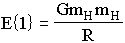12.1
where mH is the mass of the hydrogen atom. The two particles remain trapped at a distance R in this gravitational potential if the amount of electromagnetic energy emitted is equal to E{1}. The equivalent loss of mass to stabilize this interaction is equal to:12.2
Therefore, after stabilization by the emission of radiation, using equations 12.1 and 12.2, we find that the remaining mass M{1} of the pair of hydrogen atoms (at distance R) is:12.3
After the formation of the first pair of hydrogen atoms, let a new hydrogen atom fall (at a distance R) into the gravitational field produced by the new pair. The new hydrogen atom of mass mH interacts at a distance R from the pair of mass M{1} previously formed and described in equation 12.3. Using Newton's law, the gravitational energy between the pair of hydrogen atoms with mass M{1} and the individual hydrogen mH atom is:12.4
We might want to explain how the new hydrogen atom can be at an effective distance R from the previous pair of atoms. The distance R mentioned here means that the new atom is located at a distance R from the previously formed pair so that the gravitational potential between the new atom and the pair is equivalent to the potential that would exist if the previously formed pair of atoms were close together and the new atom were at a distance R from the pair. This description is supported mathematically by a theorem (used in electrostatics) which shows that the potential created at the surface of a spherical distribution of charges is the same as if all the charges were located at the center of the sphere. We will apply this same theorem here for the case of the gravitational potential of particles approaching the spherical distribution of matter forming the star.
In equation 12.4, the mass DM{2} lost after emitting thermal energy is:12.5
The total mass M{2} of the three hydrogen atoms is then:
 M{2} = M{1} + mH - DM{2} 12.612.7
Equations 12.7, 12.3 and 12.4 give:12.8
Of course, when a star is formed, the energy does not have to be emitted immediately after the addition of each individual atom. When particles are brought together, they form a hot gas in their gravitational potential which cools down later by the emission of radiation. There is no difference of energy if the radiation is emitted immediately or later.
Repeating the operation and adding a fourth hydrogen atom to the set of three atoms gives:12.9
Equations 12.8 and 12.9 give:12.1
Adding another hydrogen atom to the growing mass gives:12.11
Equations 12.10 and 12.11 give: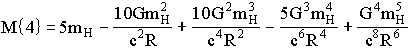12.12
Let us define:12.13
Then:
 M{4} = 5mH - 10mH2 Z + 10mH3 Z2 - 5mH4 Z3 + mH5 Z4 12.14
 M{5} = 6mH - 15mH2 Z + 20mH3 Z2 - 15mH4 Z3 + 6mH5 Z4 - mH6 Z5 12.15
The seventh hydrogen atom gives:
 M{6} = 7mH - 21mH2 Z + 35mH3 Z2 - 35mH4 Z3 + 21mH5 Z4 - 7mH6 Z5 + mH7 Z6 12.16
Going on with more individual atoms but limiting our calculations to the fourth power of mH gives:
 M{7} = 8mH - 28mH2 Z + 56mH3 Z2 - 70mH4 Z3 12.17
 M{8} = 9mH - 36mH2 Z + 84mH3 Z2 - 126mH4 Z3 12.18
 M{9} = 10mH - 45mH2 Z + 120mH3 Z2 - 210mH4 Z3 12.19
 M{10} = 11mH - 55mH2 Z + 165mH3 Z2 - 330mH4 Z3 12.2
 M{11} = 12mH - 66mH2 Z + 220mH3 Z2 - 495mH4 Z3. 12.21
The coefficients of the equations above can be generalized to give: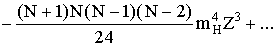12.22
For a star like the Sun, the value of N is about 1057. Then for N>>1 equation 12.22 gives: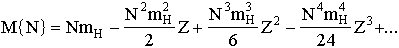12.23
which is identical to: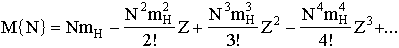12.2412.25
Let us define:
 Y = NmH 12.26
Equation 12.25 becomes:12.27
This can be written (N is so large that it can be approximated to ¥ ):12.28
We recall that Y = NmH is the total mass of the nebula that formed the star. This would be the mass of the star if there were no energy (mass) lost through radiation during the formation. M{N} is the final mass of the star made of N hydrogen atoms after taking into account the thermal energy emitted as explained above.

12.3 - Mass of a Star versus the Amount of Matter Used for Its Formation.
Equation 12.28 gives the mass of the star as a function of the amount of matter Y used to form it. Of course, when a larger amount of matter falls into the gravitational potential, thermal energy is emitted and the amount of mass lost into radiation increases. In these calculations, the value of Z (from equation 12.13) is kept constant when we study a star having a fixed radius R. Figure 12.1 shows the final mass of the star (after temperature stabilization) as a function of the total mass falling on it, using Z = 1 in equation 12.28.
We see on figure 12.1 and from equation 12.28, that for a very small amount of hydrogen atoms, the total mass of the star is almost the same as the mass of the atoms used before the formation. However, when the number of atoms accumulated in the star becomes larger, the gravitational potential acting on each newly added hydrogen atom becomes increasingly important.Figure 12.1

More energy is lost in thermal radiation after each new hydrogen atom is added. Consequently, an increasing fraction of the new mass is lost when the star becomes more massive.
Here is a numerical example obtained from equation 12.28. When the total input of mass from the nebula is 0.01 (YZ = 0.01), independently of the value of Z, about 99.5% of that mass remains in the star. For one unit (YZ = 1.0) of input mass, the final mass is 63% of the initial matter. When the input mass is ten units (YZ = 10.0), only 0.005% of the new mass is added to the star. Finally, when the amount of matter given by the nebula to form the star becomes much larger, the new mass added to the star becomes almost completely transformed into energy due to the gigantic gravitational potential. Therefore the mass of the star no longer increases when the value of YZ gets very large (as shown on figure 12.1).

12.4 - Mass of a Star versus its Radius.
Within the limits explained above, let us now consider a different way to build a star. Instead of increasing the amount of matter from outer space while forming the star at a constant radius, we use a constant number of hydrogen atoms from the nebula but all matter is contracted into a star of radius R.
When the star is initially very big, the gravitational potential at its surface is negligible. A very large star appears almost like a concentrated nebula without an intense gravitational potential. However, when the radius gets smaller, the high density star generates a much higher gravitational potential so the increase of temperature generates radiation which causes a loss of mass-energy of the shrinking star. Using equation 12.28, we can calculate the radius of the star formed from a contracting nebula containing a constant number of atoms of matter. During the decrease of the radius, the star is maintained at a relatively low temperature (of a few tens of thousand degrees), due to Planck's emission of radiation.Figure 12.2

When the total number of particles N (= Y/mH) coming from the nebula is kept constant, Z(R) becomes the variable (see equation 12.13). For Y = 1, let us calculate the residual mass of the star as a function of its radius R. After temperature stabilization, the relative mass of the star (with respect to the mass of the initial nebula) as a function of the radius R is given by equations 12.13 and 12.28. This is illustrated on figure 12.2.
We see that when the radius of the nebula (or the star) decreases, the star loses mass as electromagnetic radiation more and more rapidly.

12.5 - Maximum Mass of a Star versus Its Radius.
Let us assume now that the mass available Y is so large that the product YZ is always larger than 10. In that case, the value of the bracket in equation 12.28 reaches a maximum of 1.0. Let us substitute equation 12.13 in equation 12.28. This gives:12.29
Since the maximum value of the bracket in equation 12.29 is 1.0, the maximum value of M{N} as a function of R is:12.3
Equation 12.30 shows that the maximum mass of a star increases linearly with its radius R. Above this limit, any mass falling freely on the star reaches a kinetic energy equal to its mass so that the same amount of radiation energy is freed and there is no net increase of mass of the star. The incoming particle is totally transformed into radiation which totally escapes from the star.

12.6 - Complete Transformation of Mass into Energy.
There is another way to find the maximum mass of a star of radius R. We have seen that the gravitational energy E(Pot) of a particle of mass m at a distance R from the surface is given by: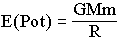12.31
We know that independently of their masses, all particles reach the same velocity when they fall from outer space to the surface of the same star. During their fall, particles acquire kinetic energy. The kinetic component of energy of a particle moving at velocity v is given by (g-1)m in the equation:
 mv = gm 12.32
where12.33
During the fall of a particle in the gravitational potential of a star, no energy is coming from outside the system. Consequently, the total energy of the falling particle remains constant during an unperturbed fall.
This result is different from the inertial acceleration of a mass absorbing energy given by an external independent source. Due to that external source of energy, the total energy of the particle increases as given by equation 12.32. However, when falling freely in a gravitational field, the kinetic energy increases at the expense of the gravitational energy of the particle.
Let us consider a particle reaching the surface of a star (of maximum mass). The velocity corresponds to g = 2 (v = 0.866c). Then the kinetic energy Ek is equal to the initial mass at rest:
 Ek = mc2 12.34
When the particle hits the surface of the star, the kinetic energy is released and emitted toward outer space (either immediately as gamma rays or later as thermal energy). When this happens, the loss of mass Dm is equal to the mass of the particle m. At the surface of the star, the kinetic energy of the particle is equal to the gravitational energy it has lost. We have:12.35
Therefore, in that limit case, the mass Mlim of the star is: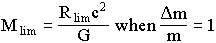12.36
Consequently, any mass falling from outer space to the distance Rlim from the star of mass Mlim will be totally annihilated into radiation. As expected, this result is identical to equation 12.30. Consequently, when the surface of the star is at such a deep gravitational potential, there is no possibility of increasing the mass of the star any further. Finally, if a particle has an initial velocity toward the star when entering the outer limits of the gravitational field, more energy will be removed from the star through radiation than the amount added by the particle. The mass of the star then decreases since more mass escapes by radiation than the amount of mass added by the particle.
Of course, near the surface of a star (which has a maximum mass), the gravitational potential is enormous so that clocks run at a very slow rate. Matter located in this extreme gravitational field will interact according to the proper parameters existing at that location. Consequently, the spectrum of the Planck radiation emitted from this deep potential will be emitted according to the local clock which runs very slowly. The spectrum will be displaced toward longer wavelengths with respect to outer space where clocks run more rapidly as explained in chapter one. However, after its emission from the location in the deep gravitational potential, light will not be redshifted again while traveling against the gravitational field as explained in chapters one and ten.
If we consider a particle reaching the ultimate potential at a distance Rlim from the center of the star, there is no possibility for it to move deeper inside that radius because there is nothing left of the particle. It would be absurd to discuss the behavior of particles at or inside that extreme radius since they no longer exist and all their energy and mass have been transformed completely into radiation.

Comparison.
This relationship for the maximum mass of a star can be compared with the Schwarzschild radius. Let us note that the Schwarzschild radius RS has an incomprehensible meaning in our context. Just as for general relativity, it is not compatible with the principle of mass-energy conservation. It is given by the relationship: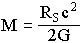12.37
12.7 - Proper Values in Extreme Gravitational Potentials.
Let us consider that an observer in outer space measures the distances between the center of a star (having the maximum mass Mlim) and different bodies stationary at different distances. Using his proper units, the outer space observer can measure the distances between the center of the star and the closest body existing around it (which is near Rlim) up to the more distant masses. However, the observers located on each of those bodies will use their proper units to make their measurements of their own distance from the center of the star. They must use these proper values in order to apply correctly the well-known physical relationships. We have seen that the absolute length of the meter is longer for an observer located closer to the star. Consequently, when measuring the same absolute radius, the number of proper meters will be smaller for the observer close to the star than for the outer space observer.

Using the equations given in chapter four, we see that when the distance from the star is large (in the Newtonian limit), the number of proper meters measured by an outer space observer is almost identical to the number obtained by an observer not too close to the star. However, when the observer is close to the extreme minimum radius Rlim, the use of the extremely dilated proper meter will give a number of proper meters approaching zero (and not Rlim(o.s.)). For this reason, physical phenomena taking place near location Rlim (using internal proper values) appear very strange to an outer space observer.
Near that location (Rlim), the Bohr and nuclear radii get very large and the corresponding energy inside particles becomes extremely small with respect to the external mechanical forces. In outer space, we are used to see internal (atomic and nuclear) forces of matter being much larger that the mechanical and gravitational forces. Near a degenerate star, nuclear forces are much weaker. This phenomenon favors reactions between particles.
Let us also recall that in the first chapters of this book, we were calculating very small relativistic interactions (i.e. Mercury precessing around the Sun). It was then enough to consider the first order of a series expansion. However, when we consider bodies with kinetic energy in a very deep gravitational potential, these approximations are no longer accurate.

12.8 - Beyond the Extreme Gravitational Potential.
Let us consider a star having a maximum mass and therefore surrounded by an extreme potential. We have seen that when an hydrogen atom gets closer to the surface of the star, its mass decreases when brought to rest and its clock slows down in the same proportion. We have seen that the same maximum gravitational potential can exist at the surface of stars having different radii. When the nucleus of this star approaches that extreme limit of gravitational potential, the number of particles forming that star approaches infinity while the mass of each atom approaches zero. The product of these two parameters approaches a constant (for a given radius) as shown in equation 12.30.
Finally, extrapolating (to a smaller radius) beyond this extreme potential, the mass of the falling hydrogen atom disappears at the same time as the clock becomes infinitely slow and finally stops running at Rlim. In fact, one can say indifferently that the clock has stopped running or that the clock has disappeared and no longer exists. Therefore clocks become infinitely slow at the same time as they disappear completely out of existence. In physics, it is absurd to study matter inside the critical radius Rlim.

12.9 - Formation of Matter in a Deep Gravitational Potential versus the Formation of Matter and Anti-Matter.
We have seen above that mass can be transformed into radiation in a deep gravitational potential without requiring a reaction between matter and anti-matter. In physics, there is another well-known mechanism transforming mass into radiation: the annihilation of a particle with its anti-particle. For example, we know that an electron and a positron can be annihilated into radiation. As expected, the corresponding inverse mechanism is also known from the interaction of photons creating a pair of matter and anti-matter. It is important to notice that the reaction of annihilation of matter with anti-matter is extremely rapid so that matter formed at the same time (and at the same location) can survive only during an extremely short time before being annihilated. Particles and anti-particles destroy each other at a very high rate. This system is quite unstable. Furthermore, since matter and anti-matter are formed simultaneously at the same location, it is ultimately improbable that they could separate out to form independent galaxies. Consequently, another mechanism of formation of matter without involving anti-matter is required to explain our universe if we want to avoid ad hoc hypotheses.

12.9.1 - Inverse Gravitational Mechanism.
We have seen in this chapter how matter falling in a deep gravitational potential is finally transformed into radiation. This mechanism cannot be maintained forever in the universe because all matter would be transformed into radiation. We have explained above how the formation of matter through the mechanism of matter and anti-matter cannot lead to the formation of huge clusters of galaxies of matter in the universe as we observe them. There must be an equilibrium between the formation and the annihilation of matter in the universe. Mass-energy conservation is not compatible with the creationist theory that claims that the universe was formed from nothing ten or fifteen billion years ago.
It is well known in physics that for every mechanism, an inverse mechanism exists. The simple absorption of radiation by matter is to some extent an intermediate mechanism of transformation of energy into mass without involving anti-matter. However, in that case, atoms become more massive but no new atoms are formed.
A simplistic description of the inverse mechanism corresponding to the annihilation of matter in a gravitational field is the following. Since radiation is emitted when atoms hit a surface located in a deep gravitational potential, we can foresee that energetic radiation hitting the surface of the same star could generate particles with sufficient kinetic energy so that they could reach the escape velocity vesc ( = 0.866c) of a star with extreme mass and be freed in outer space. Of course, other mechanisms involving gravity can be suggested but are beyond the discussion of the present book.
When matter falls into an extreme gravitational potential, it is transformed into energy without involving a reaction between matter and anti-matter. Consequently, the inverse reaction must equally correspond to the formation of matter without the creation of anti-matter. We have seen that a reaction generating matter plus anti-matter is not acceptable to explain the origin of matter in the universe, because of the extremely fast inverse reaction returning matter into radiation. We see now that a mechanism using gravity can explain the transformation of matter in the universe.
The transformation of matter into radiation (and its inverse reaction) is an extremely slow process since the time for a star to emit the thermal energy during its formation depends on its size but generally takes at least a few hundred million years. One can expect that the inverse reaction transforming radiation into neutral particles can take a few billion years before forming nebulae which later evolve into stars and later into other bodies with a very deep gravitational potential. Such mechanisms would finally form a complete cycle transforming matter into radiation and vice versa. On the average this cycle would repeat itself every ten or fifteen billion years. In such a case, after a full cycle, the information about the exact previous structure of the universe would be lost. From this mechanism, matter of the universe could be recycled periodically. During that cycle, since there would be large variations in the time taken by concentrations of masses to evolve, the universe would always look more or less the same through time. The possibility of such a mechanism becomes highly probable when taking into account the red shift mechanism taking place in our universe as demonstrated  in previous papers.

12.10 - References.
 P. Marmet, A New Non-Doppler Redshift, (Book), Physics Dept. Laval University, Québec, Canada, 64p., 1981.
also:
P. Marmet, A New Non-Doppler Redshift, Phys. Essays, 1, 24-32, 1988.
also:
P. Marmet, Redshift of Spectral Lines in the Sun's Chromosphere, IEEE, Transactions on Plasma Science: Space and Cosmic Plasma 17, 238-243, 1989.
also:
P. Marmet and Grote Reber, Cosmic Matter and the Non-Expanding Universe, IEEE, Transactions on Plasma Science, 17, 264-269, 1989.
also:
P. Marmet, Non-Doppler Redshift of Some Galactic Objects, IEEE, Transactions on Plasma Science, 18, 1, P. 56-60, 1990.

<><><><><><><><><><><><>

Chapter11 Contents    Appendix 1